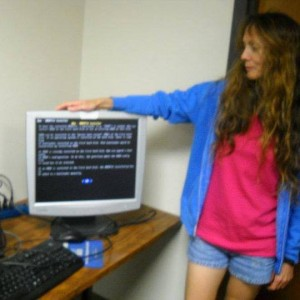Ware Repair closed August 28th, 2020

# The Mathematics Involved in 3D Game Programming

### The Mathematics Involved in 3D Game Programming

If you've played three dimensional (3D) games before, you have probably wondered - how do they do this? How do computer programmers create this amazing 3D-space illusion that shows up on the monitor?

Two-dimensional space basically allows you to travel left or right, and up or down, and any diagonal variation away from such directions, all on a flat plane, similar to the older style Super Mario Brothers game. These directions and their points are normally given coordinates from x and y axes on the coordinate plane, similar to what is taught in algebra classes.

But most modern games, and most likely the ones you play now, like Need For Speed, Halo, or Player Unknown Battleground, to name a few, involve three dimensions. Therefore, three axes are needed, the x, y, and z axes.

The other day I decided to so some research on 3D programming, whether it be for game development or other uses, such as CAD or medical diagnosing, and I came upon several books which give insight into this fascinating, yet difficult endeavor. So I thought I would share what I discovered. And, to be honest, I also wanted to study some mathematics subjects again, since this was my primary major in college and it has been a while since I delved into this area.

Also note, as mentioned in the title that this is the beginning of a series of blogs on this matter. This first blog will simply give the main outline.

So, what is involved here?

First of all, as the title of my blog implies, yes, 3D game programming is highly math-based.

Video games try to simulate actual reality, whereas computers are binary-based number crunchers. Since computers are based on numbers, 3D realms must be built mathematically (but then again, the very basis of all computer functions is mathematical, using the binary number system, with just 0s and 1s at the very core of all processing).

The main mathematical areas for 3D programming are vectors and the coordinate systems (x, y, and z axes for 3D realms), matrices, and transformations.

Vector Algebra

The first main area of math for 3D programming is vector algebra, involving vectors. Whereas a scalar quantity only has magnitude, a vector has both magnitude and direction. Vectors are usually represented as a line segment with a starting point, the tail end, and an ending point, an arrowhead. Below are a few examples shown on the x and y axes, and on x, y, and z axes as well.Vectors play an important role in computer graphics, collision detection, and physical simulation. Vectors are used to represent positions, displacements, directions, velocities, and forces, and as mentioned above, used to model physical quantities that possess both magnitude and direction. And then there are operations that can be performed on vectors, such addition and subtraction of vectors, or the dot product and cross product, but all of this will be discussed in a later blog.

Matrix Algebra

The second main area of math for 3D programming is matrix algebra, involving matrices. Matrices are used in 3D graphics to compactly describe geometric transformations, such as scaling, rotation, and translation.Matrices are also used to change the coordinates of a point or vector from one frame to another. A matrix is a rectangular array of real numbers, represented as m x n, with m rows and n columns. And as with vector algebra, there are operations that can be performed on matrices. Some examples are matrix addition, matrix multiplication, multiplying a matrix with a scalar quantity, and some other mathematical operations, again to be discussed in more depth in later blogs.

Transformations

The third main area of math for 3D programming is transformations.

This is rather the most interesting part, in my opinion. To create a 3D experience, objects need to be described in a 3D realm geometrically, which means as a collection of triangles that approximate the exterior surfaces of these objects. Check out the cute cat image below, to get some idea of this.And since these objects should not remain motionless, transformations are used. This is often referred to as transforming geometry, or geometric transformations. Some examples of geometric transformations are translation, reflection, rotation, and scaling (as can be seen below).Matrix equations can be used to transform points and vectors in 3D space.And again, I will discuss more of the details about transformations in later blogs.

3D Programming

Now, it is one thing to know the necessary mathematics to produce 3D programming, but then it is quite another thing to be able to represent this math in computer programming. Several programming languages can accommodate 3D programming, but C++ and C are the main programming languages. Windows DirectX and Windows version of C++ are often the main components for creating 3D games. I plan on later writing other blogs on this topic as well.

Sources for this article (and some great books to check out on this subject!):
Introduction to 3D Game Programming, by Frank Luna

Mathematics for 3D Game Programming and Computer Graphics, by Eric Lengyel

Essential Mathematics for Games and Interactive Applications, by James M. Van Verth and Lars M. Bishop### Diane Ware

###### Manager - Tech Support

I am co-owner of Ware Repair and enjoy working with technology, web design and development, and embedded programming, especially that which applies to robotics. I also enjoy writing blogs and sci-fi novels on my free time.